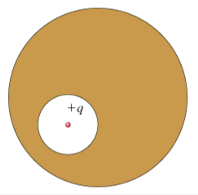# Problem: A spherical cavity is hollowed out of the interior of a neutral conducting sphere. At the center of the cavity is a point charge, of positive charge q.Now a second charge, q2, is brought near the outside of the conductor. Which of the following quantities would change?(a) The total surface charge on the wall of the cavity, qint.would changewould not change(b) The total surface charge on the exterior of the conductor, qext.would changewould not change

###### FREE Expert Solution

(a)

The total surface charge in the conductor is zero.

q + qint = 0

qint can only be changed by changing the charge enclosed in the cavity.###### Problem Details

A spherical cavity is hollowed out of the interior of a neutral conducting sphere. At the center of the cavity is a point charge, of positive charge q.Now a second charge, q2, is brought near the outside of the conductor. Which of the following quantities would change?

(a) The total surface charge on the wall of the cavity, qint.

• would change
• would not change

(b) The total surface charge on the exterior of the conductor, qext.

• would change
• would not change